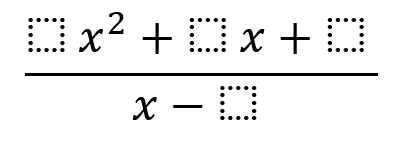# Polynomial Division Given a Remainder

Directions: Using the digits 1 to 9 at most one time each, place a digit in each box to so that the remainder when dividing the two is 14.### Hint

Is there a way to find the remainder without using long or synthetic division?